# CHEMISTRY the study of matter MATTER anything that

• Slides: 20CHEMISTRY the study of matter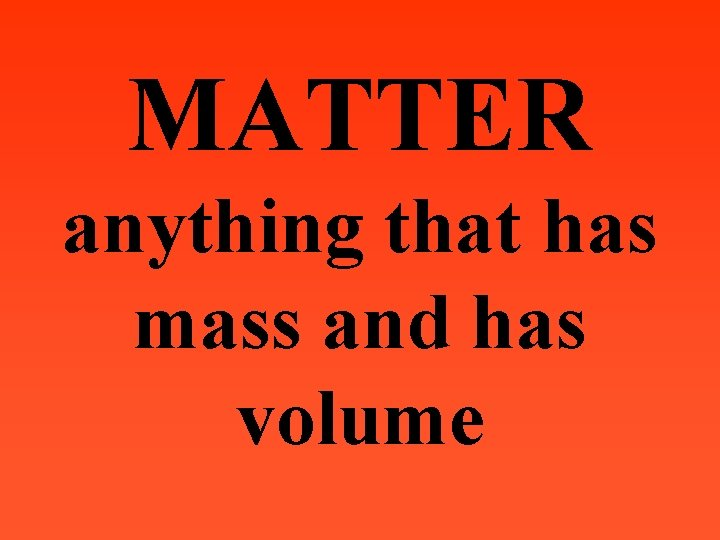MATTER anything that has mass and has volume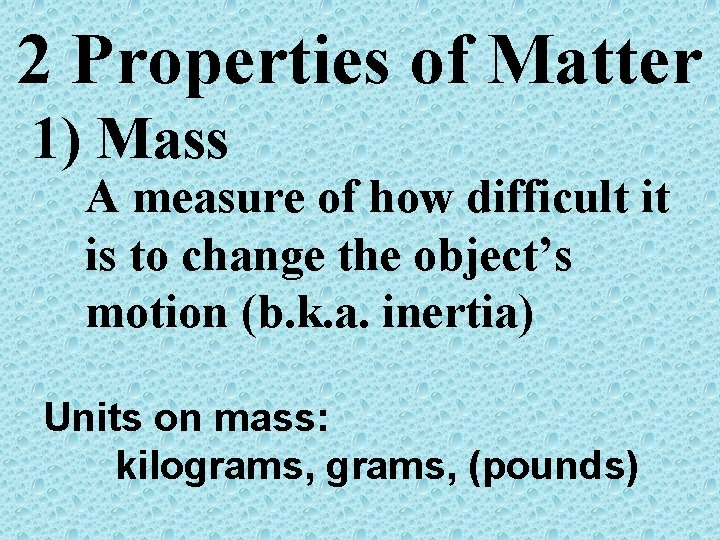2 Properties of Matter 1) Mass A measure of how difficult it is to change the object’s motion (b. k. a. inertia) Units on mass: kilograms, (pounds)Massive objects are harder to move. Thus they have more matter and more inertia Which has more inertia?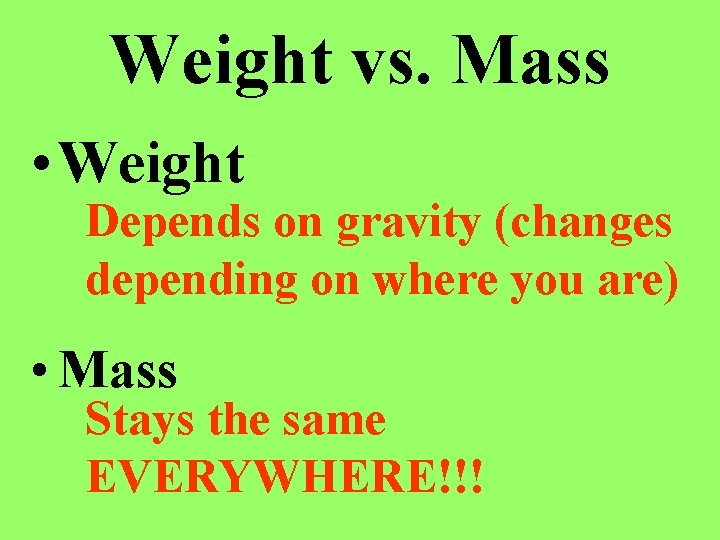Weight vs. Mass • Weight Depends on gravity (changes depending on where you are) • Mass Stays the same EVERYWHERE!!!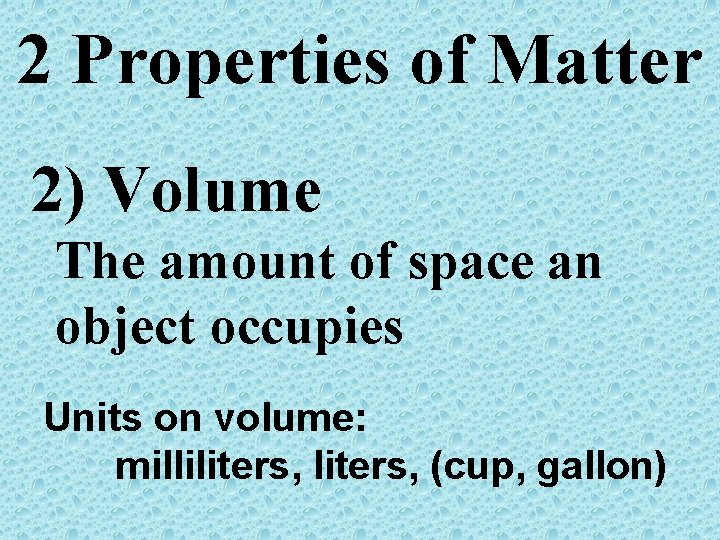2 Properties of Matter 2) Volume The amount of space an object occupies Units on volume: milliliters, (cup, gallon)An object’s volume can be found in 4 basic ways: 1. Multiplying dimensions 2 3 together (lwh, πr h, 4/3 πr ) • Volume displacement • Using a liquid measure (m. L) • As a derived unit (D=m/v)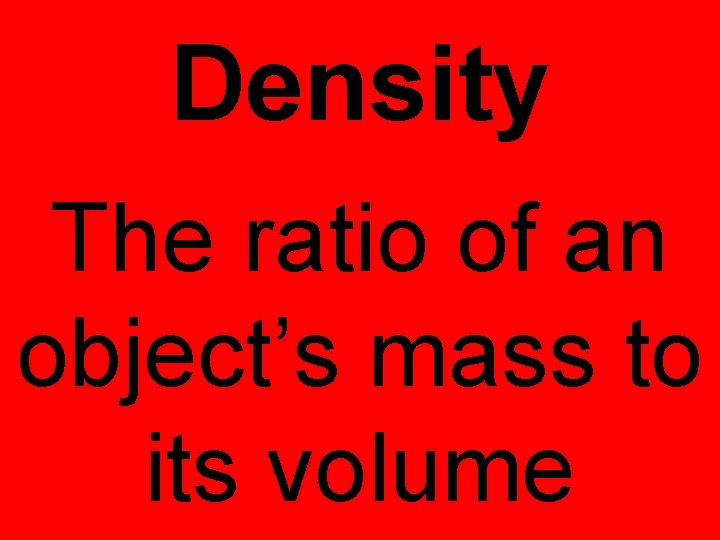Density The ratio of an object’s mass to its volumeUnits: g/m. L, 3 g/cm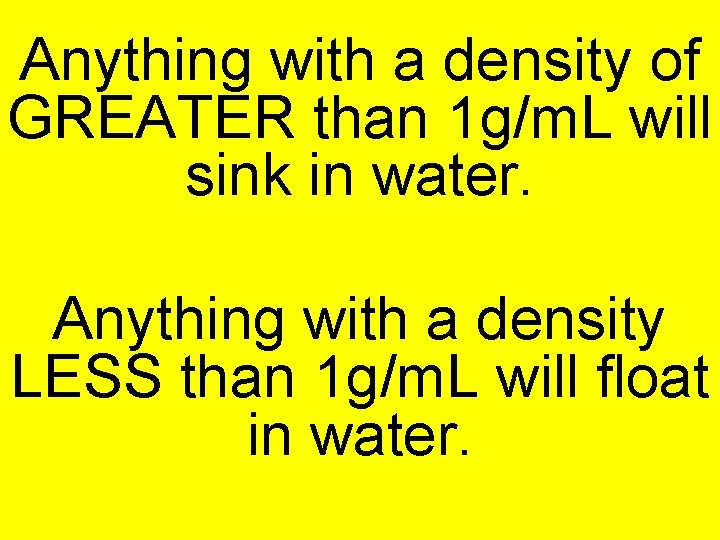Anything with a density of GREATER than 1 g/m. L will sink in water. Anything with a density LESS than 1 g/m. L will float in water.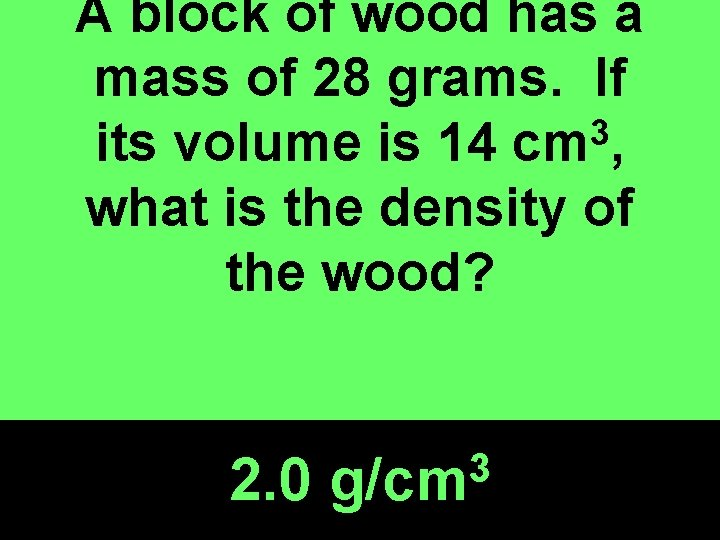A block of wood has a mass of 28 grams. If 3 its volume is 14 cm , what is the density of the wood? 2. 0 3 g/cm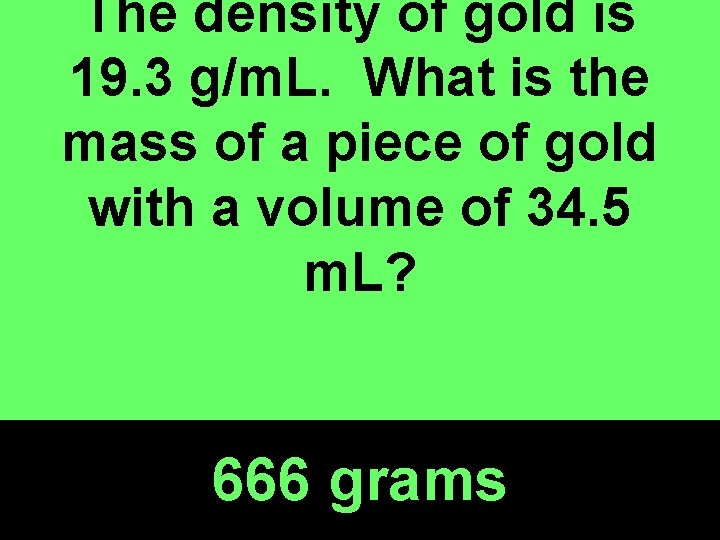The density of gold is 19. 3 g/m. L. What is the mass of a piece of gold with a volume of 34. 5 m. L? 666 grams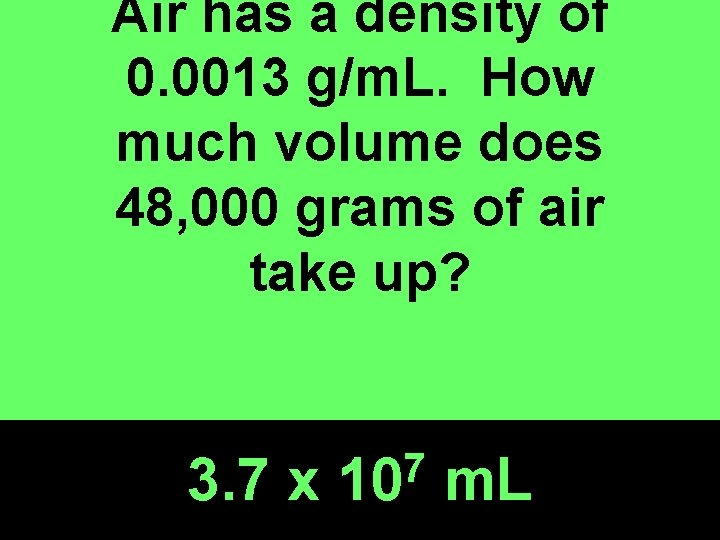Air has a density of 0. 0013 g/m. L. How much volume does 48, 000 grams of air take up? 3. 7 x 7 10 m. L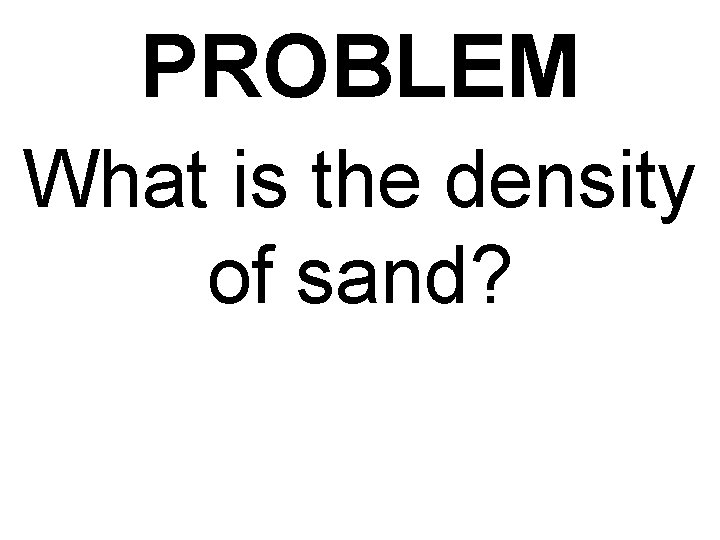PROBLEM What is the density of sand?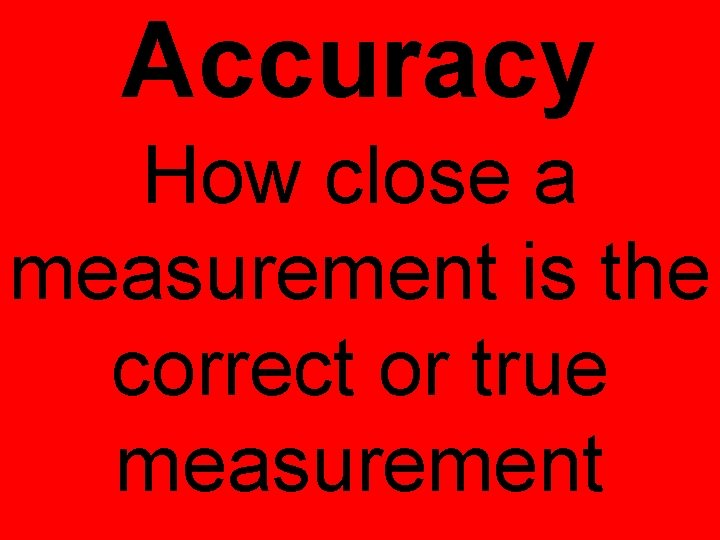Accuracy How close a measurement is the correct or true measurementPrecision How close a set of measurements are to each other (regardless of how correct they are)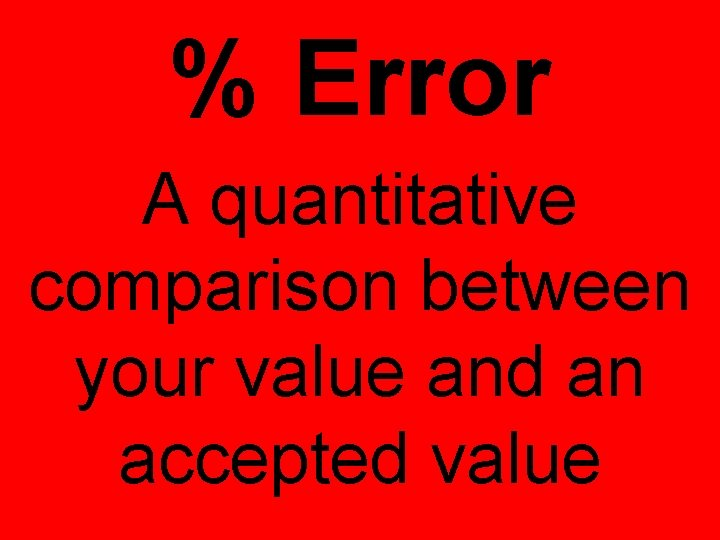% Error A quantitative comparison between your value and an accepted valueYour value for water density = 0. 88 Accepted value for water density = 1. 0 Calculate the % error in your measurement!